, 2008-11-20

# Introduction

In the previous part of this series, we talked about the importance of spatial data in our everyday lives. We talked about vector and raster data, and we explained that the current version of SQL Server 2008 focuses on two-dimensional vector data. Next, we introduced the new geography and geometry data types in SQL server 2008, and we took a quick detour into some important object-oriented principles.

In this article, we start out by taking a look at the class hierarchies for both the geometry and the geography data types in SQL Server 2008. We will focus on the concrete classes in the class diagram which are:

1. Point.
2. LineString
3. Polygon

We will also take a look at the collection classes in the object hierarchy, which allow us to store multiple instances of each type.

Once we have a good grasp on the geometry and geography class hierarchy we will dive into the details of each concrete class. We will start our investigation by looking into the details of the Point and LineString class, and we will write a T-SQL script that highlights the most important features. In the process we will take a look at the three different data formats that can be used to represent spatial data:

1. The Well-Known-Binary (WKB) format
2. The Well-Known-Text (WKT) format
3. The Geography Markup Language (GML) data format.

All of these formats are published as a standard by the Open Geospatial Consortium (OGC), which is the leading standards body for geospatial and location based services.

# The Geometry class hierarchy

## Overview

A class diagram showing the Geometry class and all of its sub-classes is shown below (note that the Geography class diagram is basically identical, with the Geography class at the root of the tree):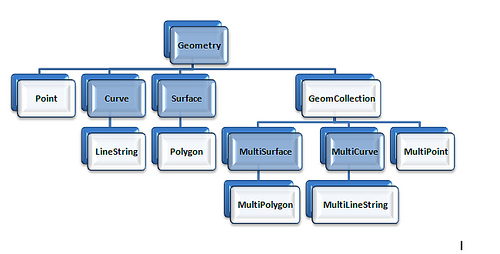The darker-blue shaded classes are abstract base classes, so they cannot be directly instantiated in the database engine. The light-blue shaded classes are the concrete classes that we can use directly in our T-SQL code:

1. Point
2. LineString
3. Polygon

Each of these single-instance classes has a collection equivalent that can contain multiple instances:

1. MultiPoint
2. MultiLineString
3. MultiPolygon

The GeomCollection class is an additional collection class that can contain a mixture of instances of any type (Points, LineStrings and Polygons).

## Supported Data Formats

All of the classes in the geometry and the geography class hierarchy can be represented by three different formats:

1. The Well-Known Binary (WKB) format. This is a byte-stream (binary) representation for a geography instance. This format is the preferred serialization format for those applications that need to store geospatial information in a compact, self-contained format.
2. The Well-Known Text (WKT) format. This is a compact, easy to read representation. Because of its user-friendly format it is the most commonly used representation for ad-hoc geospatial queries.
3. The Geography Markup Language (GML) format. This is an XML-based representation, which is best suited for including geospatial information in an XML document. This format is very useful for those applications that need to exchange geospatial information by means of an XML Web service.

All of the above formats are published as standards by the OGC. In the code samples that follow, we will be showing multiple examples of each format.

# The Point Class

## Basic Representation

A Point is a zero-dimensional object which represents a single, exact location. It always contains an X and Y coordinate, and can optionally can contain a Z (elevation) value, and a M (measure) value. The measure value is an arbitrary floating point measurement value that you can associate with the Point instance.

The sample T-SQL script (GeometryPoint.sql)illustrates some of the capabilities of the Point class. Throughout all of our demos, we will be working with a very simple table called GeometryDemo, which allows us to associate a Geometry instance with an integer ID:

```CREATE TABLE GeometryDemo( ID INT NOT NULL, Value GEOMETRY NOT NULL);
```

In the following code sample, we insert a Point instance, and then perform a select to retrieve the point instance in a number of formats:

```-- Insert a simple Point with an-- X and Y coordinateINSERT INTO GeometryDemo (ID, Value)VALUES (1, 'POINT(50 65)');
```
```-- Get the X and Y coordinates and -- the most relevant methodsSELECT ID, Value.ToString() AS WKT,  Value.STX AS [X Coordinate],  Value.STY AS [Y Coordinate], Value.STAsBinary() as [Binary Representation], Value.STAsText() as [Text Representation]FROM GeometryDemoWHERE ID = 1;
```

In the first code snippet, we use the WKT format to insert a Point with an X coordinate of 50 and a Y coordinate of 65. The WKT format for a Point simply requires you to to specify the X and Y coordinates. Note that you should NOT use a comma separator between the two, which might seem counterintuitive at first.

In the second snippet we select the instance that we just inserted. Since Value is a geometry class instance, we need to use the dot (".") notation to access properties and invoke methods on the object:

• First we invoke the ToString() method. This method will return the Well-Known Text (WKT) representation of the instance.
• Next we invoke the STX and STY properties to get the X and Y coordinates of our Point.
• We then invoke the STAsBinary() method to get the binary representation of the Point.
• Finally we invoke the STAsText() method, which will again return the WKT format of the Point.

One important note: although TSQL by itself is not case sensitive, the CLR methods and properties on the spatial data types are, so make sure that you spell the property and method names correctly! For example if you would spell "Value.STAsText()" as "Value.STasText()" you would get the following error message:

Msg 6506, Level 16, State 10, Line 34

Could not find method 'STasText' for type 'Microsoft.SqlServer.Types.SqlGeometry' in assembly 'Microsoft.SqlServer.Types'

The output of the select query is shown below: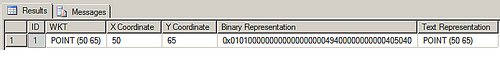From the above output, we see that the WKT representation for the Point is returned by both the ToString() and the STAsText() methods. We also see the binary WKB representation and the X and Y coordinates of the Point instance.

## Using the Z and M coordinates

As we mentioned earlier, a Point can optionally contain a Z (Elevation) coordinate and a M (Measurement) value. Note that both the Z and M values should be floats. Below is a T-SQL example:

```-- Insert a Point with an X, Y, Z and-- M coordinateINSERT INTO GeometryDemo (ID, Value)VALUES (2, 'POINT(55 70 100.2 50)');
```
```SELECT ID, Value.ToString() AS WKT,  Value.STX AS [X Coordinate],  Value.STY AS [Y Coordinate], Value.Z AS [Elevation], Value.M AS [Measure]FROM GeometryDemoWHERE ID = 2;
```

As you can see, we simply added the Z and M values in the WKT format. The output of the select query is shown below: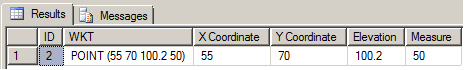## OGC-compliant methods and Microsoft Extensions

The attentive observer probably noticed that some method and properties have an ST prefix (such as STX, STY and STAsText()), while other properties and methods do NOT have this prefix (for example: Z, M and ToString()). All methods and properties that are prefixed with ST are OGC-compliant methods, while the other methods and properties are Microsoft extensions to the OGC standard.

In the documentation you will notice that the OGC standard methods and the Microsoft extensions are cleanly separated: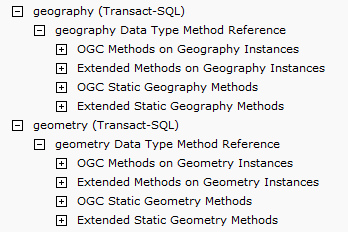In the above diagram we also see sections for static methods (both OGC and Microsoft extensions). We will take a look at static methods in a later section of this post.

## Geography Markup Language (GML)

The only format that we have not worked with so far is the Geographic Markup Language (GML), let's write a query to retrieve both points as GML:

```-- Select both Points as GML-- Note that we are NOT retrieving the Z and M-- coordinates of the second point-- because they are NOT OGC compliant!--SELECT ID, Value.AsGml() as [GML]FROM GeometryDemo;
```

The AsGml() method can be used to retrieve a geometry instance in GML format.
The output of the query is shown below: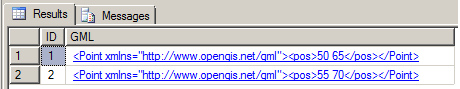The standard XML namespace for GML will have the URN: http://www.opengis.net/gml.

There is also one important note that we can make from the above output: the Z and M coordinates that we used for the second point are NOT returned in the GML! That is because they are proprietary Microsoft extensions to the OGC standard.

## Using the static Point methods

The methods and properties we have used so far were invoked on an instance of the Point class, so we used the "dot" (.) notation:

```instanceName.Property
```
```-or-
```
```instanceName.MethodCall(... method arguments here ...)
```

Instance properties and methods are always tied to a particular object instance, in this case a Point instance. Besides instance methods, the spatial data types in SQL Server 2008 also use static methods and properties. Static methods and properties are tied to the class itself, and can thus be invoked without having an instance around. You use the "::" notation in combination with the class name to invoke a static method or property in T-SQL:

```className::Property
```
```-or-
```
```className::MethodCall()
```

One frequent usage pattern for a static method is the Factory Pattern. The Factory Pattern returns a new instance of the class through a static method. This pattern is used in both geospatial types in SQL Server 2008. Below is a code example from the GeometryPoint.sql T-SQL script:

```/* ====================================================================  ===== Using the Static methods to create a Point Instance ============ ======================================================================
*/DECLARE @Pnt1 Geometry, @Pnt2 Geometry, @Pnt3 Geometry, @Pnt4 Geometry, @Pnt5 GeometryDECLARE @xmlSnippet xml;
```
```-- 1. Create a Point from an X and Y coordinate and an SRIDSET @Pnt1 = geometry::Point(30, 20, 0);
```
```-- 2. Create a Point using the Parse methodSET @Pnt2 = geometry::Parse('POINT( 90 23 56000 231)');
```
```-- 3. Create a Point using the OGC STPointFromText method-- Notice the SRID at the endSET @Pnt3 = geometry::STPointFromText('POINT(20 25)', 0)
```
```-- 4. Create a Point from GML set @xmlSnippet =  '<Point xmlns="http://www.opengis.net/gml"> <pos>34 23</pos> </Point>';SET @Pnt4 = geometry::GeomFromGml(@xmlSnippet, 0);
```
```-- 5. Null PointSET @Pnt5 = geometry::[Null];
```
```-- Now select all pointsSELECT @Pnt1.ToString() AS [From Arguments], @Pnt2.ToString() AS [From Parse], @Pnt3.ToString() as [From STPointFromText], @Pnt4.ToString() AS [From Xml], @Pnt5 AS [Null Point];
```

Following is a discussion of each static method:

• First, we use the static geometry::Point() method to create a Point instance by passing in the X, Y, Z and M coordinates.
• Next, we use the geometry::Parse() method to create a Point instance from Well-Known Text (WKT).
• Then, we use the geometry::STPointFromText() method to create a Point instance from Well-Known Text. The is the OGC equivalent of the geometry::Parse() method.
• Next, we have an example of how to create a Point instance from a Geographic Markup Language (GML) snippet, using the static geometry::GeomFromGml() method.
• Finally, we create a NULL Point instance with the static geometry::[Null] property.

The output of the select statement is shown below: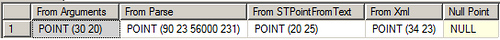As we can conclude from the above output, the Point instances were were created as expected by means of the static methods.

## The Spatial Reference Identifier

In the previous code sample, you might have noticed the "0" after the @xmlSnippet parameter:

```set @xmlSnippet =  '<Point xmlns="http://www.opengis.net/gml"> <pos>34 23</pos> </Point>';SET @Pnt4 = geometry::GeomFromGml(@xmlSnippet, 0);
```

This argument is the SRID, or the Spatial Reference Identifier. The SRID corresponds to a spatial reference system based upon the specific ellipsoid used for either flat-earth or round-earth mapping. Different projection systems exist, some of you might be familiar with the Mercator Projection that was used to create the maps that were in most classrooms in recent times. A good discussion of map projections can be found at: http://maps.unomaha.edu/Peterson/gis/notes/MapProjCoord.html.

Each projection is uniquely defined by its own SRID. You can use any projection you want, but you need to be aware that when you are comparing spatial instances using any of the methods (such as STIntersection()) you need to make sure that all objects have the same SRID otherwise you will get a run-time error!

That concludes part 2 of this series. In part three, we take a look at the LineString and the Polygon class, and we will use some visualization tools such as GeoQuery, Spatial Viewer, and SQL Server Management Studio's very own "Spatial Tab" to get a visual representation of our spatial data!

4.31 (16)

4.31 (16)

# SQL Server 2008 T-SQL Enhancements Part - IV

In the fourth installment that looks at T-SQL changes for SQL Server 2008, Ashad Ali examines how the Filestream data type works.

4.72 (40)

2010-03-26 (first published: )

19,742 reads

2017-05-01

843 reads

2014-09-19

1,940 reads

# SQL Server 2008 Spatial Index Performance

The institution I work with has decided to migrate their database system to SQL Server 2008. One of the applications uses geospatial data, which consists of millions of rows. I understand that their are indexes that can be used for geospatial data, but have not worked with them. What's the scoop on them?

2010-04-08

4,056 reads

# SQL Server 2008 T-SQL Enhancements Part - V

The fifth installment of Arshad Ali's series examines the spatial data types in SQL Server 2008. These new types allow the use of geospatial data in SQL Server applications.

4.57 (21)

2009-08-31

10,104 reads